Next: Exercises Up: Traveling Waves Previous: Doppler Effect

# Wave Propagation in Inhomogeneous Media

We saw in Section 7.6 that if a wave is normally incident at an interface between two media of substantially different impedances then a significant fraction of the wave energy is reflected. Moreover, this reflection takes place in such a manner that the net energy flux on either side of the interface is the same. On the other hand, if the two media have similar impedances then the reflected energy fraction is small. In this case, the incident and transmitted waves have the same frequency (since this property of the waves is determined by the source) but slightly different wavenumbers (assuming that the characteristic wave propagation speeds on either side of the interface are slightly different). Suppose that a wave travels though a medium whose impedance and characteristic wave propagation speed are slowly varying functions of position. We would expect such a wave to propagate through the medium without significant reflection. Moreover, the frequency of the wave should remain fixed, whilst its wavenumber slowly varies with position, in accordance with the dispersion relation. Finally, the amplitude of the wave should vary slowly with position in such a manner that the energy flux remains constant. Let us investigate further.

Suppose that the medium has a characteristic wave propagation speed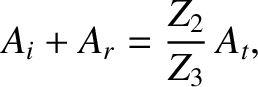, and a wave impedance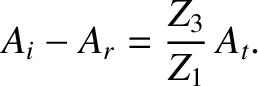. The local wave dispersion relation is assumed to take the form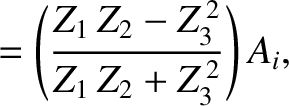(521)

Hence, at fixed wave frequency, the wavenumber varies with position as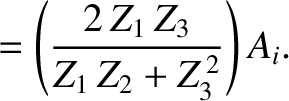(522)

If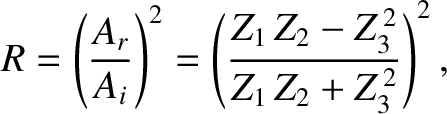is the phase of the wave then the the most general definitions of angular frequency and wavenumber are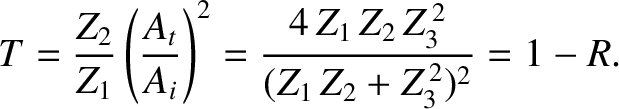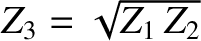(523)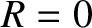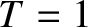(524)

respectively. (See Section 10.6.) It follows that, in the present case,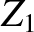(525)

Hence, the wavefunction can be written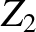(526)

where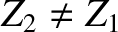is the wave amplitude. The mean energy flux associated with the wave is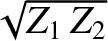(527)

(See Section 7.4.) We would expect this flux to be position independent (otherwise, energy would not be conserved), which implies that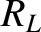. Thus, we obtain(528)

where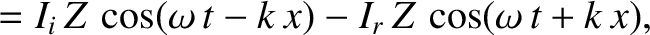is a constant. The previous expression, which is known as the WKB solution (WKB stands for Wentzel-Kramers-Brillouin), is not exact. However, it can be demonstrated that the WKB solution is a good approximation to the true solution of the inhomogeneous wave equation in the limit that the variations inandtake place on lengthscales that are much longer than the wavelength of the wave (Schiff 1955). On the other hand, the WKB solution breaks down completely if the variations inandtake place on lengthscales smaller than, or similar to, the wavelength.Next: Exercises Up: Traveling Waves Previous: Doppler Effect
Richard Fitzpatrick 2013-04-08